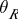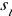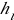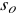# Day 152

## Reflection

• The bouncing of a wave from a barrier
• Reflection occurs with all types of waves, whether they be mechanical (requiring a medium) or non-mechanical (like electromagnetic waves)

## Diagram of Reflection

• Reflection involves the incident wave, I, the reflected wave, R, and a normal line, N. The normal is a perpendicular drawn to the barrier.•= angle between incident ray and normal;= angle between reflected ray and normal.

## Law of Reflection

• The Law of Reflection says that the angle of incidence equals the angle of reflection
• Or=• For a plane mirror, or flat mirror, it is easy to draw the reflected rays
• With light, there would be an infinite number of incident and reflected rays

## Plane Mirror Images

• When looking in a plane mirror, inspect your image. How would you describe your image with respect to left-right orientation, size and distance from the mirror?
• Answer: Your image is flipped left to right with respect to the mirror, is the same size and is the same distance behind the mirror as you are in front of the mirror. All images produced by plane mirrors are called “virtual images” because the projected rays (behind the mirror) intersect to form the image

Watch this video on mirrors.

## Concave Mirrors

Concave mirrors actually do appear to “cave in” when looking at the mirrored side (like a make-up mirror). Image production with a concave mirror depends on where the object is located in comparison to the mirror.   If you have access to a magnifying mirror or make-up mirror, try this:

• Place the mirror a good distance away from you and walk closer to it. Notice what happens to your image as you approach the mirror. Get as close as you can to the mirror.
• You should notice several things:
• When you are far away from a concave mirror, your image should be upside-down and smaller than yourself.
• At some point as you approach the mirror, you will “lose yourself” or the image will become blurry.
• As you pass this blurry point, your image will become upright and magnified.
• These mirrors are also called “converging mirrors“.

## Convex Mirrors

• Also called “diverging mirrors
• Convex mirrors curve in the opposite direction from a concave mirror.
• When looking at a convex mirror, the center protrudes outward.

With the previous concave mirror example, the “blurry” point is the focal point of the mirror. Images do not form when the object is at the focal point because the rays are parallel to each other, they do not converge or diverage, therefore there is no real or virtual point of intersection.

Think of a curved mirror as an arc on the outside of a circle.   Now, continue this arc around to complete a full circle.

• The center of the circle is called the center of curvature.
• The distance from this point to the mirror is called the radius of curvature, R.
• Half of this distance, R, to the mirror is the focal length, f.
• Therefore, R = 2f.
• Notice that this explains why f is negative for a convex mirror (it is actually inside the curve) and positive for a concave mirror (where f is outside the curve).

## The Math of Image Formation

The mirror (and lens) equations are:

• 1/f = 1/+ 1/AND/= –/• f = focal length
•= image distance (to mirror)
•= object distance (to mirror)
•= height of image
•= height of object

## Sign Rules for the Mirror Equations

• f is positive for a converging (concave) mirror; negative for a diverging (convex) mirror
• A negativemeans the image is inverted (upside-down); positive means upright
• A negativemeans the image is virtual (formed from projected rays, more info to follow)
• A positivemeans the image is real (formed from reflected rays that physically cross, more info to follow)

(source)